Future Post Random Variables in Julia compared to MATLAB/R/STATA/Mathematica/Python

Published:

This post compares the way random variables are handled in Julia/MATLAB/R/STATA/Mathematica/Python. It was inspired by Bruce Hansen’s recent textbook which compares statistical commands in Matlab/R/STATA on page 114. This post will focus on the main methods for working with random variables in a language: e.g. Distributions.jl is the flagship Julia package for random variables, MATLAB’s internal distributions, Base R, Base STATA, Mathematica, and Python’s SciPy.

1: Tables Comparing Syntax

CDF:

RVJuliaMATLABBase RSTATAMathematicaPython SciPy
$N(0,1)$cdf(Normal(0,1),x)normcdf(x)pnorm(x)normal(x)CDF[NormalDistribution[0, 1],x]norm.cdf(x)
$\chi^2_{r}$cdf(Chisq(r),x)chi2cdf(x,r)pchisq(x,r)chi2(r,x)CDF[ChiSquareDistribution[r],x]chi2.cdf(x, r)
$t_r$cdf(TDist(r),x)tcdf(x,r)pt(x,r)1-ttail(r,x)CDF[StudentTDistribution[r],x]t.cdf(x, r)
$F_{r,k}$cdf(FDist(r,k),x)fcdf(x,r,k)pf(x,r,k)F(r,k,x)CDF[FRatioDistribution[r,k],x]f.cdf(x, r, k)
$D(\theta)$cdf(D(θ),x)Dcdf(x,θ)pD(x,θ)?CDF[D[θ],x]D.cdf(x,θ)

Inverse Probabilities (quantiles):

RVJuliaMATLABBase RSTATAMathematicaPython SciPy
$N(0,1)$quantile(Normal(0,1),p)norminv(p)qnorm(p)invnormal(p)Quantile[NormalDistribution[],p]norm.ppf(p)
$\chi^2_{r}$quantile(Chisq(r),p)chi2inv(p,r)qchisq(p,r)invchi2(r,p)Quantile[ChiSquareDistribution[r],p]chi2.ppf(p, r)
$t_r$quantile(TDist(r),p)tinv(p,r)qt(p,r)invttail(r,1-p)Quantile[StudentTDistribution[r],p]t.ppf(p, r)
$F_{r,k}$quantile(FDist(r,k),p)finv(p,r,k)qf(p,r,k)invF(r,k,p)Quantile[FRatioDistribution[r,k],p]f.ppf(p, r, k)
$D(\theta)$quantile(D(θ),p)Dinv(p,θ)qD(p,θ)invD(p,θ)Quantile[D[θ],p]D.ppf(p,θ)

Other Properties:

PropertyJuliaMATLABBase RSTATAMathematicaPython SciPy
cdfcdf(D(θ),x)Dcdf(x,θ)pD(x,θ)?CDF[D[θ],x]D.cdf(x,θ)
pdf/pmfpdf(D(θ),x)Dcdf(x,θ)dD(x,θ)?PDF[D[θ],x]D.pdf(x,θ)
quantilequantile(D(θ),p)Dinv(p,θ)qD(p,θ)invD(p,θ)Quantile[D[θ],p]D.ppf(p,θ)
randomrand(D(θ),N)Dinv(p,θ)rD(N)invD(p,θ)RandomVariate[D[θ],N]D.ppf(p,θ)
meanmean(D(θ))---Mean[D[θ]]-
entropyentropy(D(θ))-----
fitfit(D, data)---FindDistributionParameters[data,D]-

2: Random Variables as Types

A key distinction between the way the packages above handle random variables is that in Julia and Mathematica a random variable is itself a type. On the other hand e.g. in R you cannot refer to the underlying randomv variable, you can only compute properties such as chi2cdf(x,r).

General syntax in Julia:
Distributions.jl distinguishes between a Random Variable’s parameters and property variables. A random variable is a type such as Chisq(r) or D(θ). A property of a random variable such as CDF or mean is (typically) a functional which takes random variable as its argument along with any necesarry property specific variables.
Note: some properties don’t have any arguments such as mean(D(θ)).
Note: the fit(D, data) function requires a distribution type without parameters D as opposed to D(θ).

3: Random Variables in Distributions.jl

In general a random variables package does three things:

• Creates random variables: built-in/fit/transform
• Sample random variables
• Compute properties: probabilities/moments/cumulants/entropies etc

Here is an overview of current features:

1. Creating Random Variables:
• Built in random variables: D(θ), Chisq(r), FDist(r,k) etc
• Combining and transforming random variables:
• Mixture models: MixtureModel([Normal(0,1),Cauchy(0,1)], [0.5,0.5])
• Truncated random variables: Truncated(Cauchy(0,1), 0.25, 1.8)
• Convolution of random variables: convolve(Cauchy(0,1), Cauchy(5,2))
• Cartesian product of random variables: product_distribution([Normal(),Cauchy()])
• Other packages for creating random variables: AlgebraPDF.jl etc
2. Sampling: rand(D(θ),N), rand(Cauchy(0,1), 100)
3. Fitting:
• parametric: fit(D, data)
• non-parametric: fit(Histogram, data)
4. Other properties: property(D(θ)) or property(D(θ),x) where θ is the vector of distribution parameters and x is the vector of property variables.
• example: d=LogNormal()
• mean(d), median(d), mode(d), var(d), std(d)
• skewness(d), kurtosis(d), entropy(d)
• pdf(d, 2), cdf(d, 2), quantile(d, .9), gradlogpdf(d, 2)
• Most properties above are implemented in closed form. There are POC tools from numerical expectation etc
Distributions.expectation(LogNormal(), cos) computes $E[cos(X)]$ where $X\sim LogNormal(0,1)$.

4: Future and other and general tranformations of random variables

Numerical vs Symbolic: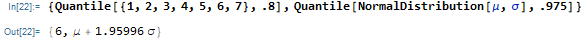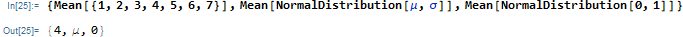I discussed the following examples on Discourse.
Distributions.jl currently doesn’t operate on transformations of random variables. Mathematica can handle transformations of a distribution when it can solve the problem symbolically.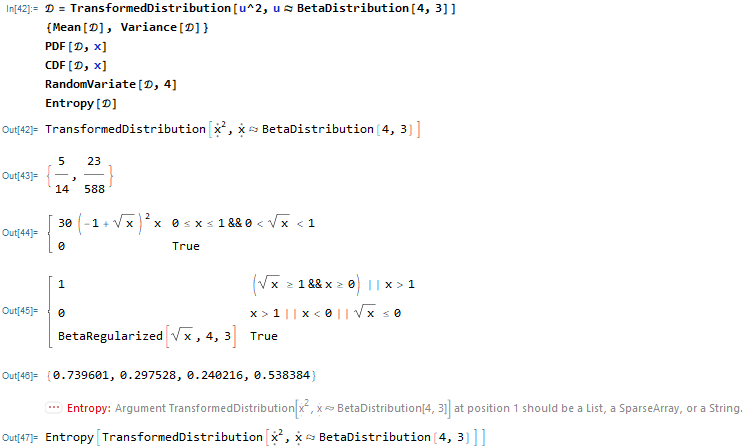Now consider the same distribution with symbolic parameters BetaDistribution[α,β]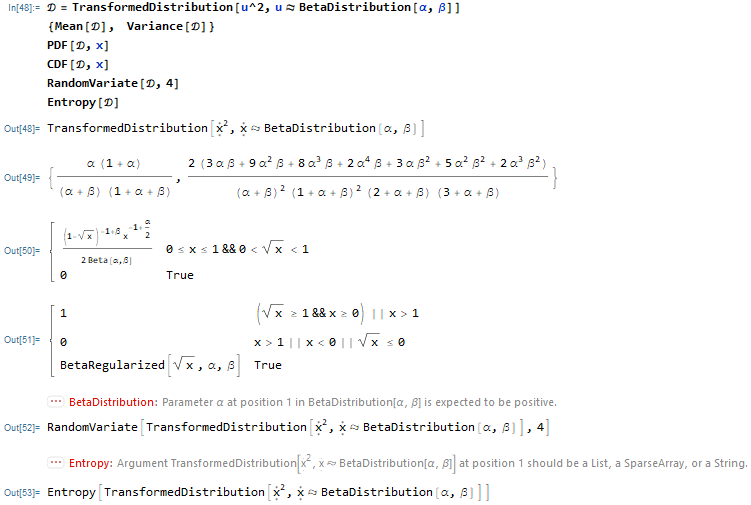From paper:
Type hierarchy

1. Sampling interface
2. Distribution interface and types
R equivalent p-d-q-r in Julia: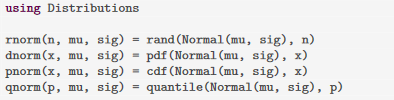3. Distribution fitting and estimation
parametric: fit(D, data)
non-parametric: fit(Histogram, data)
4. Modeling mixtures of distributions

The table below adds Julia, Mathematica, and Python.

Python: https://github.com/QuantEcon/rvlib
R: https://github.com/alan-turing-institute/distr6
Compare syntax: https://hyperpolyglot.org/scripting